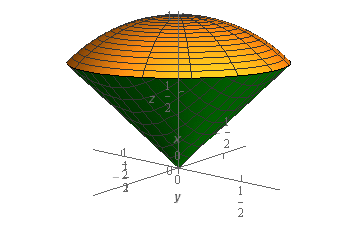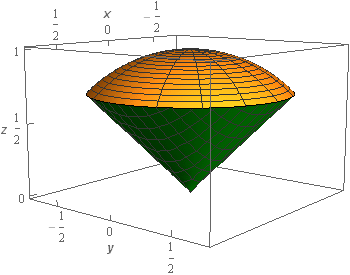Paul's Online Notes
Home / Calculus III / Multiple Integrals / Triple Integrals in Spherical Coordinates
Show Mobile Notice Show All Notes Hide All Notes
Mobile Notice
You appear to be on a device with a "narrow" screen width (i.e. you are probably on a mobile phone). Due to the nature of the mathematics on this site it is best views in landscape mode. If your device is not in landscape mode many of the equations will run off the side of your device (should be able to scroll to see them) and some of the menu items will be cut off due to the narrow screen width.

### Section 15.7 : Triple Integrals in Spherical Coordinates

3. Evaluate $$\displaystyle \iiint\limits_{E}{{3z\,dV}}$$ where $$E$$ is the region inside both $${x^2} + {y^2} + {z^2} = 1$$ and $$z = \sqrt {{x^2} + {y^2}}$$.

Show All Steps Hide All Steps

Start Solution

Okay, let’s start off with a quick sketch of the region $$E$$ so we can get a feel for what we’re dealing with.We’ve given the sketches with a set of “traditional” axes as well as a set of “box” axes to help visualize the surface and region.

In this case we’re dealing with the region below the sphere of radius 1 (the orange surface in the sketches) that is inside (i.e. above) the cone (the green surface in the sketches).

Show Step 2

Now, since we are integrating over a portion of a sphere it makes sense to use spherical coordinate for the integral.

The limits for $$\rho$$ and $$\theta$$ should be pretty clear as those just correspond to the radius of the sphere and to how much of the sphere we get by rotating about the $$z$$-axis.

The limits for $$\varphi$$ however aren’t explicitly given in any way but we can get them from the equation of the cone. First, we know that, in terms of cylindrical coordinates, $$\sqrt {{x^2} + {y^2}} = r$$ and we know that, in terms of spherical coordinates, $$r = \rho \sin \varphi$$. Therefore, if we convert the equation of the cone into spherical coordinates we get,

$\rho \cos \varphi = \rho \sin \varphi \hspace{0.25in} \to \hspace{0.25in} \tan \varphi = 1 \hspace{0.25in} \to \hspace{0.25in} \varphi = \frac{\pi }{4}$

So, the equation of the cone is given by $$\varphi = \frac{\pi }{4}$$ in terms of spherical coordinates. Because the region we are working on is above the cone we know that $$\varphi$$ must therefore range from 0 to $$\frac{\pi }{4}$$.

The limits are then,

$\begin{array}{c} \displaystyle 0 \le \varphi \le \frac{\pi }{4}\\ 0 \le \theta \le 2\pi \\ 0 \le \rho \le 1\end{array}$ Show Step 3

Plugging these limits into the integral and converting to spherical coordinates gives,

\begin{align*}\iiint\limits_{E}{{3z\,dV}} & = \int_{0}^{{\frac{\pi }{4}}}{{\int_{0}^{{2\pi }}{{\int_{0}^{1}{{\left( {3\rho \cos \varphi } \right)\left( {{\rho ^2}\sin \varphi } \right)\,d\rho }}\,d\theta }}\,d\varphi }}\\ & = \int_{0}^{{\frac{\pi }{4}}}{{\int_{0}^{{2\pi }}{{\int_{0}^{1}{{3{\rho ^3}\cos \varphi \sin \varphi \,d\rho }}\,d\theta }}\,d\varphi }}\end{align*}

Don’t forget to convert the $$z$$ into spherical coordinates and also don’t forget that $$dV = {\rho ^2}\sin \varphi \,d\rho \,d\theta \,d\varphi$$ and so we’ll pick up a couple of extra terms when converting the $$dV$$ to spherical coordinates.

Show Step 4

Okay, now all we need to do is evaluate the integral. Here is the $$\rho$$ integration.

\begin{align*}\iiint\limits_{E}{{3z\,dV}} & = \int_{0}^{{\frac{\pi }{4}}}{{\int_{0}^{{2\pi }}{{\left. {\left( {\frac{3}{4}{\rho ^4}\cos \varphi \sin \varphi } \right)} \right|_0^1\,d\theta }}\,d\varphi }}\\ & = \int_{0}^{{\frac{\pi }{4}}}{{\int_{0}^{{2\pi }}{{\frac{3}{4}\cos \varphi \sin \varphi \,d\theta }}\,d\varphi }}\end{align*} Show Step 5

Next let’s do the $$\theta$$ integration.

\begin{align*}\iiint\limits_{E}{{3z\,dV}} & = \int_{0}^{{\frac{\pi }{4}}}{{\left. {\left( {\frac{3}{4}\theta \cos \varphi \sin \varphi } \right)} \right|_0^{2\pi }\,d\varphi }}\\ & = \int_{0}^{{\frac{\pi }{4}}}{{\,\frac{3}{2}\pi \cos \varphi \sin \varphi \,d\varphi }}\end{align*} Show Step 6

Finally, we’ll do the $$\varphi$$ integration.

\begin{align*}\iiint\limits_{E}{{3z\,dV}} & = \int_{0}^{{\frac{\pi }{4}}}{{\,\frac{3}{4}\pi \sin \left( {2\varphi } \right)\,d\varphi }}\\ & = \left. {\left( {\, - \frac{3}{8}\pi \cos \left( {2\varphi } \right)\,} \right)} \right|_0^{\frac{\pi }{4}} = \require{bbox} \bbox[2pt,border:1px solid black]{{\frac{3}{8}\pi }}\end{align*}

We used the double angle formula for sine to reduce the integral to something that we could quickly do.

Not that, in this case, because the limits of each of the integrals were all constants we could have done the integration in any order we wanted to.